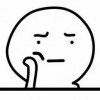2018-03-11 20:21:16

# Code:

#include<cstring>
#include<cstdio>
#include<queue>
using namespace std;
struct point
{
int x,y;
}t;
queue<point> q;
int a,vis,s;
int n,m,u;
int Find(int x)
{
if(s[x]==x)
return s[x];
return s[x]=Find(s[x]);
}
void Union(int x,int y)
{
s[Find(y)]=s[Find(x)];
return ;
}
void turn(int x)//预处理坐标转换（用空间换时间）
{
for(int i=1;i<=x;i++)
for(int j=1;j<=x;j++)
u[i][j]=(i-1)*n+j;
return;
}
int bfs(int x,int y)
{
int ans=0,k=0;
point p;
p.x=x;
p.y=y;
q.push(p);
while(!q.empty())
{
p=q.front();
q.pop();
t[++k].x=p.x;
t[k].y=p.y;
if(vis[p.x][p.y])
{
ans+=vis[p.x][p.y];
continue;
}
ans++;
if(!vis[p.x-1][p.y]&&p.x>1&&a[p.x-1][p.y]!=a[p.x][p.y])
if(Find(u[p.x-1][p.y])!=Find(u[p.x][p.y]))
{
p.x--;
q.push(p);
p.x++;
Union(u[p.x-1][p.y],u[p.x][p.y]);
}
if(!vis[p.x+1][p.y]&&p.x<n&&a[p.x+1][p.y]!=a[p.x][p.y])
if(Find(u[p.x+1][p.y])!=Find(u[p.x][p.y]))
{
p.x++;
q.push(p);
p.x--;
Union(u[p.x+1][p.y],u[p.x][p.y]);
}
if(!vis[p.x][p.y-1]&&p.y>1&&a[p.x][p.y-1]!=a[p.x][p.y])
if(Find(u[p.x][p.y-1])!=Find(u[p.x][p.y]))
{
p.y--;
q.push(p);
p.y++;
Union(u[p.x][p.y-1],u[p.x][p.y]);
}
if(!vis[p.x][p.y+1]&&p.y<n&&a[p.x][p.y+1]!=a[p.x][p.y])
if(Find(u[p.x][p.y+1])!=Find(u[p.x][p.y]))
{
p.y++;
q.push(p);
p.y--;
Union(u[p.x][p.y+1],u[p.x][p.y]);
}
}
for(int i=1;i<=k;i++)
vis[t[i].x][t[i].y]=ans;
vis[x][y]=ans;
return ans;
}
int main()
{
memset(vis,0,sizeof(vis));
scanf("%d%d",&n,&m);
for(int i=1;i<=n*n;i++)
s[i]=i;
turn(n);
char c;
scanf("\n");
for(int i=1;i<=n;i++)
{
for(int j=1;j<=n;j++)
{
c=getchar();
a[i][j]=c-'0';
}
scanf("\n");
}
int x,y;
for(int i=1;i<=m;i++)
{
scanf("%d%d",&x,&y);
printf("%d\n",bfs(x,y));
}
return 0;
}

• star
首页